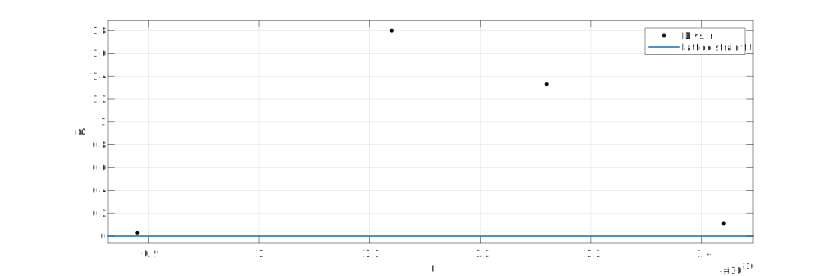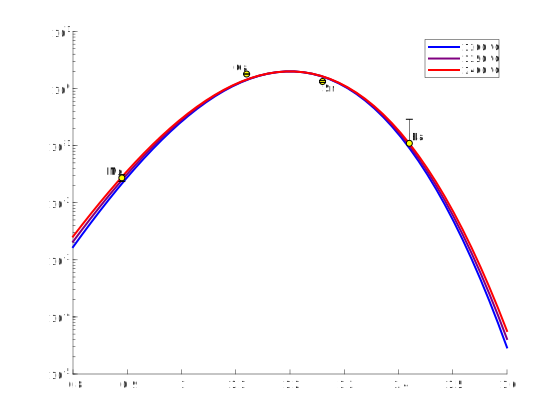# fitting a parabola giving unreasonable answer

22 views (last 30 days)
Michael Phillips on 11 Apr 2019
Commented: Michael Phillips on 12 Apr 2019
Hello,
I'm trying to fit a parabola to 4 data points using the following equation:
y = a.*exp(((-4.*pi.*b.*6.022e23)./(8.314.*1623)).*(((c./2.*(c-x).^2) - (1/3.*(c-x).^3))));
I'm getting an unreasonable result, which looks like this:I think the equation is correct because I copy and pasted it from a function that employs it to create this graph, which models the same points:John D'Errico on 12 Apr 2019
Edited: John D'Errico on 12 Apr 2019
But your model is not a parabola. It is a nasty to compute exponential thing. (Nasty in double precision arithmetic.)
Seems confusing. I'd suggest your problem is the huge dynamic range of the parameters. That gets the solver in trouble.
b = [2;-1.15e21;1.2e-10];

Clay Swackhamer on 12 Apr 2019
Two things: I changed your independent values (r) to something that is not so small. Second, I made your equation more simple. I tried it with your original values but it didn't work for me. Hopefully this gets you off to a good start.
%Data
r = [0.89;1.12;1.26;1.42];
D = [0.027322404;1.798850575;1.33;0.11];
%Set up the fit
ft = fittype('a*r^2+b*r+c', 'independent', 'r');
opts = fitoptions('Method', 'NonlinearLeastSquares');
opts.Display = 'Off';
opts.StartPoint = [0.2, 0.2, 0.3];
%Conduct the fit
[fitresult, gof] = fit(r, D, ft, opts);
%Evaluate the function for plotting
a = fitresult.a;
b = fitresult.b;
c = fitresult.c;
r_model = linspace(min(r), max(r), 100); %create 100 points to evaluate the model on
D_model = a*r_model.^2+b*r_model+c;
%Make plots
plot(r, D, 'bo', 'markerSize', 6) %plot the data
hold on
plot(r_model, D_model, 'LineWidth', 2, 'Color', 'r') %plot the model
leg = legend('Data', 'Model');
leg.FontSize = 14;Michael Phillips on 12 Apr 2019
yep no problem!

### Categories

Find more on Descriptive Statistics in Help Center and File Exchange

R2018b

### Community Treasure Hunt

Find the treasures in MATLAB Central and discover how the community can help you!

Start Hunting!